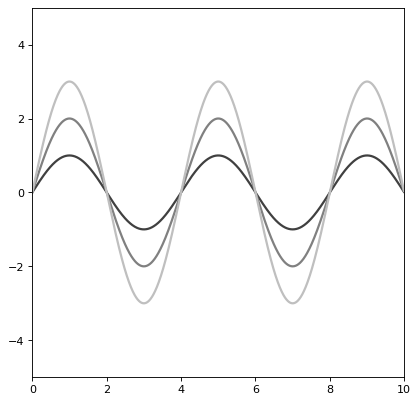# Sine1D¶

class astropy.modeling.functional_models.Sine1D(amplitude=1, frequency=1, phase=0, **kwargs)[source]

One dimensional Sine model.

Parameters
amplitudefloat

Oscillation amplitude

frequencyfloat

Oscillation frequency

phasefloat

Oscillation phase

Other Parameters
fixeda dict, optional

A dictionary {parameter_name: boolean} of parameters to not be varied during fitting. True means the parameter is held fixed. Alternatively the fixed property of a parameter may be used.

tieddict, optional

A dictionary {parameter_name: callable} of parameters which are linked to some other parameter. The dictionary values are callables providing the linking relationship. Alternatively the tied property of a parameter may be used.

boundsdict, optional

A dictionary {parameter_name: value} of lower and upper bounds of parameters. Keys are parameter names. Values are a list or a tuple of length 2 giving the desired range for the parameter. Alternatively, the min and max properties of a parameter may be used.

eqconslist, optional

A list of functions of length n such that eqcons[j](x0,*args) == 0.0 in a successfully optimized problem.

ineqconslist, optional

A list of functions of length n such that ieqcons[j](x0,*args) >= 0.0 is a successfully optimized problem.

Notes

Model formula:

$f(x) = A \sin(2 \pi f x + 2 \pi p)$

Examples

import numpy as np
import matplotlib.pyplot as plt

from astropy.modeling.models import Sine1D

plt.figure()
s1 = Sine1D(amplitude=1, frequency=.25)
r=np.arange(0, 10, .01)

for amplitude in range(1,4):
s1.amplitude = amplitude
plt.plot(r, s1(r), color=str(0.25 * amplitude), lw=2)

plt.axis([0, 10, -5, 5])
plt.show()


(png, svg, pdf)Attributes Summary

 amplitude frequency input_units This property is used to indicate what units or sets of units the evaluate method expects, and returns a dictionary mapping inputs to units (or None if any units are accepted). param_names phase

Methods Summary

 evaluate(x, amplitude, frequency, phase) One dimensional Sine model function fit_deriv(x, amplitude, frequency, phase) One dimensional Sine model derivative

Attributes Documentation

amplitude
frequency
input_units

This property is used to indicate what units or sets of units the evaluate method expects, and returns a dictionary mapping inputs to units (or None if any units are accepted).

Model sub-classes can also use function annotations in evaluate to indicate valid input units, in which case this property should not be overridden since it will return the input units based on the annotations.

param_names = ('amplitude', 'frequency', 'phase')
phase

Methods Documentation

static evaluate(x, amplitude, frequency, phase)[source]

One dimensional Sine model function

static fit_deriv(x, amplitude, frequency, phase)[source]

One dimensional Sine model derivative